# Circle calculation - calculation of radius, diameter, circumference and area

Here, everything revolves around the circle: definitions, formulas and calculations for the radius, diameter, circumference and area of the circle with different size units round off the topic. With the help of the circle calculator, you can calculate the most significant circle properties, i.e., radius, diameter, circumference and area of a circle. In the help text's of the circle calculator, each conversion for the individual circle properties is derived using the appropriate circle formula.

## Definition of a Circle

A circle is a geometric figure and is defined as the set of all points on a plane that have the same distance to a point M, the centre of the circle. This distance of each point on the circle line or on the circle edge to the centre of the circle is called the radius. Mathematically, the circle is a curve and thus one-dimensional. However, the circle is usually interpreted as a two-dimensional surface or circular disc, which is enclosed by the circle line.

The basic properties of a circle include the radius, the diameter of the circle, the circumference of the circle and the area of the circle. The calculation of these circle properties and their conversion using circle formulae can be conveniently carried out with the Circle Calculator and will be discussed in more detail below.

### What is the number Pi?

In connection with calculations and formulas for the circle and the calculation of circles, we repeatedly encounter Pi or the Greek letter π. The circle number Pi corresponds, regardless of the size of a circle, to the exact ratio of the circumference of the circle to the diameter. Pi has an infinite number of decimal places and starts with 3.1415926. The circumference of a circle is therefore always around 3.14 times larger than its diameter.

## Calculate Circle: Formulas

• Diameter: d = 2 × r
• Radius: r = ½ × d
• Circumference: C = 2 × r × π or C = d × π
• Area: A = r² × π or A = (d² × π)/4 = ¼ × d² × π

## Input 'help' for the Circle Calculator to calculate radius, diameter, circumference and area

The calculator for circle calculation contains several input fields, which are explained in more detail below:

### Size unitSelect the appropriate size unit here to display it for the individual input fields. You can select mm, cm, dm, m, km, in, ft, yd or mi.

And this is how the calculator works: You can fill in any input field below. The remaining values are then calculated and filled in automatically using the formula for the circle calculation. The help texts for radius, diameter, circumference and area always contain the current derivation of the current calculation. As soon as you change one of the values again, a new calculation of the remaining values and an update of the help texts for the derivation of the new calculation are carried out.Please enter the radius to calculate the diameter, circumference and area of the circle. The 'help texts' of the calculated circle properties will be updated to include the corresponding circle formula as well as the derivation of the calculation from the entered radius to the respective property.

### DiameterPlease enter the diameter to calculate the radius, the circumference and the area of the circle. The help texts of the calculated circle properties are then updated, which contains the associated calculation formula as well as the calculation derivation from the entered diameter to the respective property.

### CircumferencePlease enter the circumference to calculate the radius, the diameter and the area of the circle. The help texts of the calculated circle properties are updated and each contains a concrete derivation of the current calculation, i.e. from the circumference to the radius, to the diameter or to the circle area.

### AreaPlease enter an area to calculate the radius, diameter and circumference of the circle. Similarly when calculating the radius, diameter and circumference, their help texts are updated so that the derivation of the conversion in each case can be understood.

### SketchThe sketch shows you the selected circle property. This gives you an idea of how the radius, diameter, circumference and area of the circle are defined. The display changes as soon as you click on an 'input' field for the circle properties or select a corresponding help button.

## The Radius of a Circle

As already described, a circle is defined as the set of all points in the plane with an equal distance to a point M, the centre of the circle. This distance from the centre of the circle to the edge of the circle is known as the radius.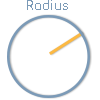The radius r or radius is the distance between the centre point M of a circle and the circle line.

## Diameter of Circle - Definition and Formula

### Definition of Circle Diameter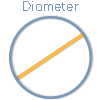The diameter of a circle d or ⌀ is the length of the connecting distance of two points of the circle line passing through the circle centre M.

The diameter is thus the greatest possible distance between two points on the circular line.

### Formula for the diameter of a circle

The diameter d corresponds to twice the radius r

d = 2 × r

## Perimeter of a Circle - Definition and Formula

### Definition of CircumferenceThe circumference C is the length of the circular line.

### Formel for Circumference

The circumference of a circle C is calculated by multiplying the diameter d by the number Pi (π = 3.1415...).

C = d × π

## Area of a Circle - Definition and Formula

### Definition of area of a circle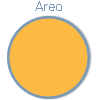The area of a circle A is the area enclosed by the circular line.

### Formula for area of a circle

The area of the circle A is calculated by multiplying the square of the radius r by Pi (π = 3.1415...).

A = r² × π

#### Video showing the derivation of the formula for the area of a circle

Here is another video on the topic 'Circles, Circumference and Area' by Math Antics. In the video, the formula for the area of a circle is derived by dividing a circle into many sectors, which are then joined together to form a rectangle. Using the radius and half the circumference of the circle, the area of this rectangle and ultimately, the circle can then be derived.

If the application does not load, it is because you are using our site without advertising and tracking. You are welcome to agree to the use of cookies to activate the application..

## Examples of Circle Calculation

The four circle properties described on this page - radius, diameter, circumference and area - can all be calculated by specifying a single value of the other property in each case. If you want to calculate the area of a circle, for example, this can be done using one of the other three properties in each case, i.e. using the radius, the diameter or the circumference.

We therefore show you below three examples for calculating the radius, the diameter, the circumference and the area of a circle using the other three circle properties in each case

## 1. Calculate the Radius of a Circle

The following three examples show the calculation of the radius using the circle properties diameter, circumference and area.

### How do you calculate the radius from the diameter?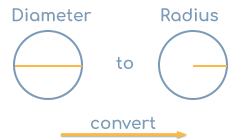Given is a circle with a diameter d of 20 in. We are looking for the radius r.

#### Calculation

The radius r is equal to half the diameter d, so r = d / 2.

Substituting the 20 in chosen in the example for the diameter d, the radius of the circle is r =  20 in / 2 = 10 in.

### How do you calculate the radius from the circumference?Given is a circle with circumference C of 30 in. We are looking for the radius r.

#### Calculation

The formula for the circumference is C = 2 × r × π. When we convert this formula to r, we get r = C / π / 2, which is half the circumference divided by Pi (π = 3.1415...), thus r = C / π / 2.

If we substitute the 30 in chosen in the example for the circumference C, the radius of the circle is r = 30 in / π / 2 = 4.77 in.

### Video for calculating the radius from the circumference

Here is another video on 'Calculating the Circumference of a Circle Given the Radius' by Mr J: The video also shows how to convert the formula for the circumference of a circle to r in order to calculate the radius based on a given circumference. Starting at 3:09, two more examples of the conversion follow.

If the application does not load, it is because you are using our site without advertising and tracking. You are welcome to agree to the use of cookies to activate the application..

### How do you calculate the radius from the area of the circle?Given is a circular area A of 100 in². We are looking for the radius r.

#### Calculation

The general formula for the circular area A is A = r² × π. If we convert this formula to r, the radius r is equal to the square root of the result of circular area A divided by Pi (π = 3.1415...), thus r = A / π.

If we insert the 100 in² chosen in the example for the area A, the radius of the circle is r = 100 in / π = 5.64 in.

### Video for calculating the radius from the circular area

For illustration purposes, here is another video on the topic of 'Find the radius' by Brian McLogan: Here, too, the conversion of the area formula of a circle to r is explained, so that the corresponding radius can be calculated for a given circular area.

If the application does not load, it is because you are using our site without advertising and tracking. You are welcome to agree to the use of cookies to activate the application..

## 2. Calculate the Diameter of a Circle

The following examples show the calculation of the diameter using the circle properties radius, circumference and area.

### How to calculate the diameter from the radius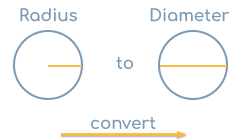Given is a circle with radius r of 10 in. We are looking for the diameter d.

#### Calculation

The diameter d corresponds to twice the radius r, i.e. d = 2 × r.

If the 10 in chosen in the example is used for the radius r, the diameter of the circle is d = 2 × 10 in = 20 in.

### How do you calculate the diameter from the circumference?Given is a circle with the circumference C of 30 in. We are looking for the diameter d.

#### Calculation

The formula for the circumference is C = d × π. If we convert this formula to d, the diameter d is equal to the circumference divided by Pi (π = 3.1415...), thus d = C / π.

If we substitute the 30 in chosen in the example for the circumference C, the diameter of the circle is d = 30 in / π= 9.55 in.

### How do you calculate the diameter from the area of the circle?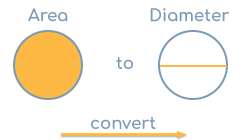Given is a circle with the area A of 100 in². We are looking for the diameter d.

#### Calculation

The general formula for the area of the circle A is A = r² × π. Since the diameter d corresponds to twice the radius r, the formula therefore is A = (d / 2)² × π. If we convert this formula to d, the diameter d is equal to twice the square root of the area of the circle A divided by Pi (π = 3.1415...), thus d = 2 × A / π.

If we insert the 100 in² chosen in the example for the area A, the diameter of the circle is d = 2 × 100 in / π = 11.28 in.

## 3. Calculate the Circumference of a Circle

The following examples illustrate the calculation of the circumference of a circle using radius, diameter and circular area.

### How do you calculate the circumference of a circle from the radius?Given is a circle with the radius r of 10 in. We are looking for the circumference C.

#### Calculation

The circumference C corresponds to twice the radius r multiplied by the number Pi (π = 3.1415...), thus C = 2 × r × π.

If we insert the 10 in chosen in the example for the radius r, the circumference of the circle is C = 2 × 10 in × π = 62.83 in.

### How do you calculate the circumference from the diameter?Given is a circle with a diameter d of 20 in. We are looking for the circumference C.

#### Calculation

The circumference C corresponds to the diameter d multiplied by the number Pi (π = 3.1415...), thus C = d × π.

If we substitute the 20 in chosen in the example for the diameter d, the circumference of the circle is C = 20 in × π = 62.83 in.

### How do you calculate the circumference of a circle from its area?Given is a circular area A of 100 in². We are looking for the circumference C.

#### Calculation

The general formula for the circular area A is A = r² × π. Using this formula, the radius r can now be calculated by first converting the formula to r. The radius r is equal to the square root of the circular area A divided by Pi (π = 3.1415...), thus r = A / π.

If we insert the 100 in² chosen in the example for the area A, the radius of the circle is r = 100 in / π = 5.64 in.

Using the radius calculated in this way, the general calculation formula for the circumference can now be used: The circumference C is equal to twice the radius r multiplied by the number Pi (π = 3.1415...), thus C = 2 × r × π.

If we insert the previously calculated value for the radius r, the circumference of the circle is C = 2 × 5.64 in × π = 35.45 in.

## 4. Calculate the Area of the Circle

The following examples illustrate the calculation of the circular area using radius, diameter and circumference.

### How do you calculate the area of a circle from the radius?Given is a circle with a radius r of 10 in. We are looking for the area of the circle A.

#### Calculation

The area of the circle A is equal to the square of the radius, i.e., r² multiplied by the number Pi (π = 3.1415...), and therefore A = r² × π.

If the 10 in chosen in the example is used for the radius r, the area of the circle is as follows A = (10 in)² × π = 314.16 in².

### How do you calculate the area of a circle from the diameter?Given is a circle with a diameter d of 20 in. We are looking for the area of the circle A.

#### Calculation

The area A is equal to half the diameter squared, i.e. (d / 2)² multiplied by the number Pi (π = 3.1415...) and thus A = (d / 2)² × π.

If we substitute the 20 in chosen in the example for the diameter d, the area of the circle is A = (20 in / 2)² × π = 314.16 in².

### How do you calculate the area of a circle from the circumference?Given is a circle with a circumference C of 30 in. We are looking for the area of the circle A.

#### Calculation

The area A is derived from the circumference, for example, by first determining the radius of the circle r on the basis of the circumference. Then you can use the common formula for the area of a circle, namely A = r² × π.

The calculation of the radius based on the circumference is already derived in the example for the conversion from circumference to radius and is 4.77 in.

Now if we insert the value for the radius r calculated here on the basis of the circumference of 30 in chosen in the example, namely 4.77 in, the area of the circle is A = (4.77 in)² × π = 71.62 in².

## Source information

As source for the information in the 'Circle' category, we have used in particular:

## Last update on February 20, 2023

The pages of the 'Circle' category were last editorially reviewed by Michael Mühl on February 20, 2023. They all correspond to the current status.

### Previous changes on October 24, 2022

• 24.10.2022: Publication of the section Calculate Circle together with associated texts.
• Editorial revision of all texts in this category